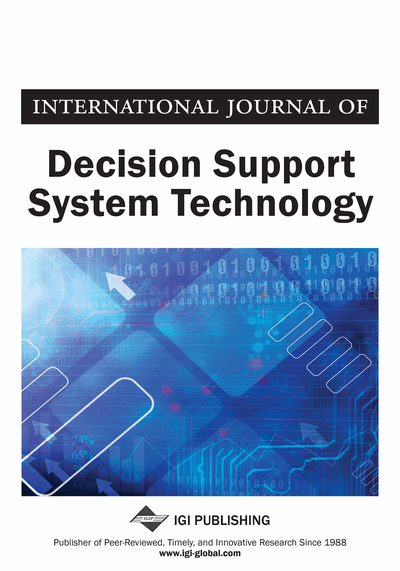# Distributed Manufacturing Networks: Optimization via Preprocessing in Decision Guidance Query Language

Nathan Egge (George Mason University, USA), Alexander Brodsky (George Mason University, USA) and Igor Griva (George Mason University, USA)
Copyright: © 2012 |Pages: 18
DOI: 10.4018/jdsst.2012070103
OnDemand PDF Download:
Available
\$37.50
No Current Special Offers

## Abstract

The authors consider optimization problems expressed in Decision Guidance Query Language that may involve linear arithmetic constraints, as well as finite domain and binary variables. They focus on Distributed Manufacturing Network optimization problems in which only a part of the problem is dynamic, i.e., the demand for the output products in a manufacturing network, whereas the rest of the problem is static, i.e., the connectivity graph of the assembly processes and the cost functions of machines. The authors propose the Online Decomposition Algorithm based on offline preprocessing that optimizes each static problem component for discretized values of shared constraint variables, and approximate the optimal aggregated utility functions. The Online Decomposition Algorithm uses the pre-processed approximated aggregated cost functions to decompose the original problem into smaller problems, and utilizes search heuristics for the combinatorial part of the problem based on the pre-processed look-up tables. They also conduct an initial experimental evaluation which shows that the Online Decomposition Algorithm, as compared with Mixed Integer Linear Programming, provides an order of magnitude improvement in terms of both computational time and the quality of found solutions for a class of problems for which pre-processing is possible.
Article Preview
Top

## Introduction

In this paper we focus on the problem of optimizing a distributed manufacturing network (DMN), in which a number of products need to be manufactured. Each product can be produced by one or more assembly node processes, where each assembly is composed of several machines working in parallel, as shown in Figure 1. For the required demand of the output products, a decision must be made on how much should be produced by each DMN assembly node, which machines should be on and their output level. Operation of each machine has associated cost, which is a function of the machine output level. Typically, the cost function has an S shape, indicating less efficient operation at the edges of its operational range, and more efficient operation in its ”sweet spot”. In turn, each DMN assembly node requires sub-products as its input, and those in turn are produced by other assemblies. Thus the overall DMN may involve multiple layers of DMN assembly nodes. An important question is how to make production decisions that minimize the total production cost.

The state-of-art implementation of decision optimization solutions, such as the DMN problem, involves Mathematical and Constraint Programming (MP and CP), used for decision optimization, i.e., finding values for control variables that maximize or minimize an objective within given constraints. While software developers find database programming mostly intuitive, they typically do not have the mathematical expertise necessary for MP and CP. In addition, much investment has already been spent on database applications. Clearly, it is desirable to leverage this investment when building decision optimization applications.

To bridge the gap, in Brodsky, Bhot, Chandrashekar, Egge, and Wang (2009) we introduced a Decision Guidance Query Language (DGQL) and system, following the Decision Guidance Management System framework of Brodsky and Wang (2008). In Brodsky, Egge, and Wang (2011) we provided DGQL formal syntax and semantics, a reduction of DGQL to the standard mathematical programming (MP) formulation, and a proof of its soundness and completeness. DGQL makes a step toward making decision optimization easier, especially in applications where database technology has been heavily used.

DGQL provides a query-like abstraction for expressing decision optimization problems so that database programmers would be able to use it without prior experience in MP and, more importantly, they would be able to reuse the queries already built into existing applications. A decision optimization problem in DGQL is written as a “regular” database program, i.e., a sequence of SQL views and accompanying integrity constraints, together with some annotation of which database table column needs to be decided by the system (i.e., variables) and toward what goal (i.e., optimization objective). Here, existing queries in SQL can be directly used. Essentially, DGQL allows users to write an optimization problem as if writing a database program in SQL in a forward manner.

The challenge in the DGQL approach, however, is how to execute the “inverse” decision optimization based on a “forwardly” expressed code. This is done in Brodsky, Bhot, Chandrashekar, Egge, and Wang (2009) and Brodsky, Egge, and Wang (2011) by encoding the DGQL queries as an MP problem, solving it using an MP solver (such as ILOG CPLEX MILP solver) and then deriving the solution to the DGQL original problem. This approach was demonstrated to be comparable in efficiency to equivalent MP formulations that were expertly crafted and solved using a Mixed Integer Linear Programming (MILP) solver.

However, for optimization problems such as the DMN problem considered in this paper, even when only linear arithmetic constraints are used, the DGQL compiler generates a Mixed Integer Linear Programming (MILP) formulation (Schrijver, 1998), which involves a large number of binary decision variables. Thus, for this family of optimization problems, standard algorithms for MILP such as the branch and bound method are highly inefficient.

## Complete Article List

Search this Journal:
Reset
Open Access Articles
Volume 13: 4 Issues (2021): 1 Released, 3 Forthcoming
Volume 12: 4 Issues (2020)
Volume 11: 4 Issues (2019)
Volume 10: 4 Issues (2018)
Volume 9: 4 Issues (2017)
Volume 8: 4 Issues (2016)
Volume 7: 4 Issues (2015)
Volume 6: 4 Issues (2014)
Volume 5: 4 Issues (2013)
Volume 4: 4 Issues (2012)
Volume 3: 4 Issues (2011)
Volume 2: 4 Issues (2010)
Volume 1: 4 Issues (2009)
View Complete Journal Contents Listing